Programmable Logic Device and Programmable Logic Array are terms for integrated circuits that can be programmed to perform complex functions.  A design is programmed into the device by blowing hundreds of micro fuses.

I assume the reader knows an AND gate from an OR gate.  Here we will talk about programmable logic ICs.  The IC below includes eight different logic functions.  To use TTL logic this will take an 74LS08 AND gate, 74LS04 Inverter, 74LS02 NOR gate….etc. Using programmable logic we can push all this logic into one IC.  The designer can greatly reduce the printed circuit real estate used.  A design change may take only minutes.  No soldering iron needed.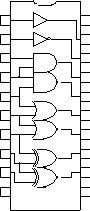The best part of programmable logic is “its programmability”.  To make this IC I typed this into my computer.  Thirty seconds later I had a custom IC.

PIN 19 = PIN 1

PIN 18 = /PIN 2

PIN 17 = PIN 3 * PIN 4

/PIN 16 = PIN 4 * PIN 5

PIN 15 = PIN 5 + PIN 6

/PIN 14 = PIN 6 + PIN 7

PIN 13 = PIN 7 :+: PIN 8

/PIN 12 = PIN 8 :+: PIN 9

The input of the inverter can be any input pin, just as the output can be on any output pin.  This greatly eases the printed circuit board layout.

To understand programmable logic arrays look at a simplified schematic of a PLD.  Signal from the two input pins and the inverse of these signal are fed into a bank of fuses F1 through F8.  The AND gates are OR-ed together which drives the output pin.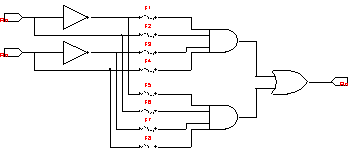By blowing open certain fuses all many gate combinations can be programmed into this simple PAL.

 Fuses closed Fuses open symbol IN AB OUT C Buffer C = A F2 F5,6,7,8 F1,3,4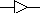0 1 0 1 Iverter C =1/A F1 F5,6,7,8 F2,3,4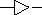0 1 1 0 AND C=A*B F2,4 F5,6,7,8 F1,300 01 10 11 0 0 0 1 NAND 1/C=A*B F1,7 F2,3,4 F5,6,8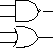00 01 10 11 1 1 1 0 OR C=A+B F2,8 F1,3,4 F5,6,7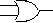00 01 10 11 0 1 1 1 NOR 1/C=A+B F1,3 F5,6,7,8 F2,4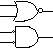00 01 10 11 1 0 0 0 XOR C=A:+:B F1,4,6,7 F2,3,5,8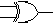00 01 10 11 0 1 1 0 XNOR 1/C=A:+:B F1,3,6,8 F2,4,5,7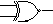00 01 10 11 1 0 0 1

This design is based around a PAL10H8 which is not in production now.  You can see the data sheet at my web site.  It is much like my simple schematic but with 10 input pins and 8 output pins.  Each AND gate has 20 inputs or 20 fuses.  Why 20 fuses?  There are 10 inputs and the inverse of those inputs.

# LATCH

A simple latch can be made.  When L is high data flows from D to Q.  When L in low the data on Q is held independent of the D input.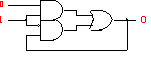# AND OR Combination Cell

Schematics of the inside of a PAL are drawn like this.  Each “X” is a fuse and a diode.  This method is much simpler than drawing each fuse.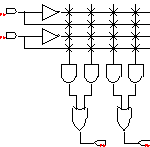See 10H8

# I/O Cell

A pin can be set to input, output or dynamically programmed as I/O.  The fuse block is far larger than shown here.  All AND gates can connect to all of the ten inputs and all of the I/O pins.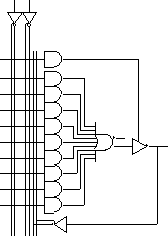See 16L8

# Registered Cell

A registered cell consists of a D-flip flop at the output of AND OR combinational logic.  This cell is used to make state machines, counters and shift registers.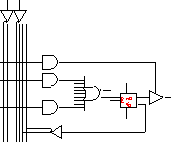See 16R8

# PAL

Many things have changed cense the first programmable logic device.  Fuses have been replaced with flash memory allowing easy erasure and reprogramming.  There used to be hundreds (more or less) of different kinds of PLD.  The industry moved to parts like the 16V8 and 22V10 which can replace almost all of the older parts.  Programmable Array Logic is basically more of the same but bigger and more flexible.  To get a data sheet for the 22V10 go to digikey.com and search for 22V10.  Then down load the PDF file.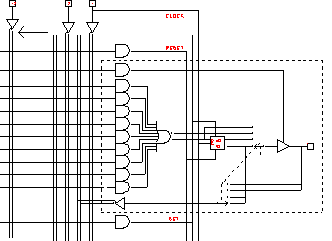Simplified schematic of a 22V10 PLD

There are 12 input pins and 10 I/O pins.  The dotted box is repeated ten times.  Each AND gate has 44 inputs.  Every input, it’s inverse and every I/O pin and it’s inverse are fed into the fuse block.  The bank of fuses total roughly 6,000.

The D flip flops have a common rising edge clock, asynchronous reset and asynchronous set controls.  Each input/output pin has a tri-state buffer controlled by one AND gate.  Each logic block is individually programmed to use/not use the flip flop.  There are four options selected by two fuses.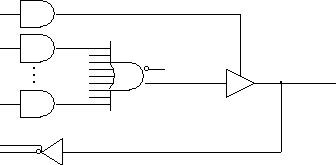Option one is a simple AND OR array with tri-state buffer.  The pin can be fixed as input or fixed as output, dynamically set as input or output.  To be pin-out compatible with older parts I used the inverse of Pin 11 to drive some or all of the tri-state controls.  The I/O pin is fed back into the fuse block.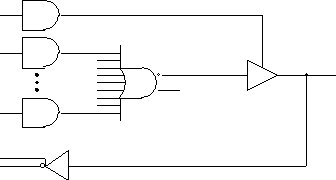The second option is AND NOR array.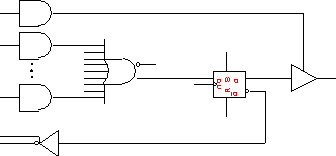Option 3 uses a D- flip flop.  Data  on D is latched on the rising edge of Pin 1 (clock).  Note the feedback now is from the FF and not the I/O pin.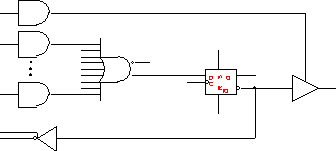Option 4 inverts the output but not the feedback.

Program

I use my EPROM programmer to program PLDs.  In programming mode a PLD looks like a small EPROM or FLASH memory.  In the case of the 22V10 the first 44 locations in memory are the 44 fuses for the first AND gate.  Each AND gate uses the next 44 fuses.  Location 0 connects Pin1 while location 1 connects 1/Pin1.  Location 4 and 5 connects Pin2 and 1/Pin2.

Software:

I have used PALASM, ABEL and CUPL.  A quick search of the Internet shows that several universities are still using PALASM.  There are sites for the students to down the software and tutorials.  A DOS PLD development program is not exciting but the price is right!  I have put PALASM on my web site, see the end of the article.

Learn by example:

PATTERN  A

REVISION 3.0

AUTHOR   J.ENGINEER

DATE     10/01/04

CHIP  DECODER  PAL16L8

;---------- PIN Declarations -----

PIN  1  CLK  COMBINATORIAL ; INPUT

PIN  2  X    COMBINATORIAL ; INPUT

PIN  3  Y    COMBINATORIAL ; INPUT

PIN  4  Z    COMBINATORIAL ; INPUT

PIN  5  GO   COMBINATORIAL ; INPUT

PIN  6  STOP COMBINATORIAL ; INPUT

PIN  10 GND                ; INPUT

PIN  12 A    COMBINATORIAL ; OUTPUT

PIN  13 B    COMBINATORIAL ; OUTPUT

PIN  14 C    COMBINATORIAL ; OUTPUT

PIN  15 D    COMBINATORIAL ; OUTPUT

PIN  16 E    COMBINATORIAL ; OUTPUT

PIN  17 F    COMBINATORIAL ; OUTPUT

PIN  18 G    COMBINATORIAL ; OUTPUT

PIN  19 H    COMBINATORIAL ; OUTPUT

PIN  20 VCC                ; INPUT

EQUATIONS

/A = /X * /Y * /Z

A.TRST = GO * /STOP

/B = /X * /Y *  Z

B.TRST = GO * /STOP

/C = /X *  Y * /Z

C.TRST = GO * /STOP

/D = /X *  Y *  Z

D.TRST = GO * /STOP

/E =  X * /Y * /Z

E.TRST = GO * /STOP

/F =  X * /Y *  Z

F.TRST = GO * /STOP

/G =  X *  Y * /Z

G.TRST = GO * /STOP

/H =  X *  Y *  Z

H.TRST = GO * /STOP

Most software have the same requirements.

Define what type of IC.   PAL16L8

Define PINs as to input, output or IO.

Give PINs names.  (optional)

Define EQUATIONS.

/H = X and Y and Z

3 input NAND gate

# Counter

EQUATIONS

;EIGHT BIT COUNTER

Q1:=/Q1                    ; OUT=1/Q1

Q2:=(Q2 :+: /Q1)          ;

Q3:=(Q3 :+: (/Q2*/Q1))

Q4:=(Q4 :+: (/Q3*/Q2*/Q1))

Q5:=(Q5 :+: (/Q4*/Q3*/A2*/Q1))

Q6:=(Q6 :+: (/Q5*/Q4*/Q3*/A2*/Q1))

Q7:=(Q7 :+: (/Q6*/Q5*/Q4*/Q3*/A2*/Q1))

Q8:=(Q8 :+: (/Q7*/Q6*/Q5*/Q4*/Q3*/A2*/Q1))

The equation Q1:= causes a flip flop to be used.  The D input of the flip flop is the inverse of the Q output.  This will cause the output to change every clock.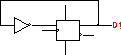Q2: toggles when Q1=0.  This will cause the output to change every other clock.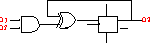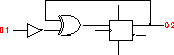Q3: holds it’s present value when D1 and D2 is not 00.  When D1,2 is 00 then Q3 changes state.

# What Really Happened

A 22V10 does not have an XOR function.  So what really happened!  I looked at the fuse map and found this.

If Q1=1 then the FF will hold.  If Q1=0 then the FF will toggle.  This is a T flip flop.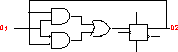# Output file

The software takes equations like /H=X*Y*Z and produces program files like this.  Each “0” or “1” represents a fuse open or closed.  In this case each line is for one AND gate.

L0000 01111111111111111111111111111111*

L0032 11111111111111111111111111111101*

L0064 00000000000000000000000000000000*

L0096 00000000000000000000000000000000*

L0128 00000000000000000000000000000000*

L0160 00000000000000000000000000000000*

L0192 00000000000000000000000000000000*

L0224 00000000000000000000000000000000*

L0256 11111111111111111111111111111101*

L0288 11110111111111111111111111111111*

L0320 11111111111111111111111101111111*

L0352 11111111111111111111111111110111*

Partial listing for a PAL16V8.

# CPLD

Very quickly:  A CPLD is a Complex Programmable Logic Device.  Basically a CPLD is four to ten 22V10s in one package.  Here we are talking about 100s of logic elements.

See MAX 3000 and MAX 5000

# Next Month FPGA

Next month, FPGAs with 100,000 logic elements, memory and a CPU!  This is where the big boys play!